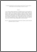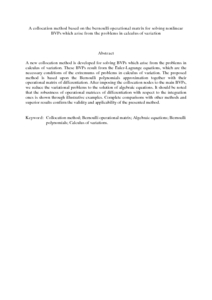# A collocation method based on the bernoulli operational matrix for solving nonlinear BVPs which arise from the problems in calculus of variation

## Citation

Tohidi, Emran and Kilicman, Adem (2013) A collocation method based on the bernoulli operational matrix for solving nonlinear BVPs which arise from the problems in calculus of variation. Mathematical Problems in Engineering, 2013. art. no. 757206. pp. 1-9. ISSN 1024-123X

## Abstract

A new collocation method is developed for solving BVPs which arise from the problems in calculus of variation. These BVPs result from the Euler-Lagrange equations, which are the necessary conditions of the extremums of problems in calculus of variation. The proposed method is based upon the Bernoulli polynomials approximation together with their operational matrix of differentiation. After imposing the collocation nodes to the main BVPs, we reduce the variational problems to the solution of algebraic equations. It should be noted that the robustness of operational matrices of differentiation with respect to the integration ones is shown through illustrative examples. Complete comparisons with other methods and superior results confirm the validity and applicability of the presented method.Preview
PDF (Abstract)
A collocation method based on the bernoulli operational matrix for solving nonlinear BVPs which arise from the problems in calculus of variation.pdfView Item